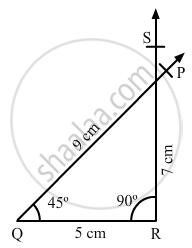Share

# Construct a Quadrilateral Pqrs, in Which ∠Q = 45°, ∠R = 90°, Qr = 5 Cm, Pq = 9 Cm and Rs = 7 Cm. - Mathematics

Course
ConceptConstructing a Quadrilateral When Two Adjacent Sides and Three Angles Are Known

#### Question

Construct a quadrilateral PQRS, in which ∠Q = 45°, ∠R = 90°, QR = 5 cm, PQ = 9 cm and Rs = 7 cm.

#### Solution

Steps of construction:

Step I: Draw QR = 5 cm .

Step II: Construct ∠PQR = 45° at Q .

Step III : With Q as the centre and radius 9 cm, cut off QP = 9 cm .

Step IV: Construct ∠QRS = 90° at R .

Step V : With R as the centre and radius 7 cm, cut off RS = 7 cm .

Since, the line segment PQ and RS intersect each other, the quadrilateral cannot be constructed .Is there an error in this question or solution?

#### APPEARS IN

RD Sharma Solution for Mathematics for Class 8 by R D Sharma (2019-2020 Session) (2017 to Current)
Chapter 18: Practical Geometry (Constructions)
Ex. 18.4 | Q: 6 | Page no. 11
Solution Construct a Quadrilateral Pqrs, in Which ∠Q = 45°, ∠R = 90°, Qr = 5 Cm, Pq = 9 Cm and Rs = 7 Cm. Concept: Constructing a Quadrilateral - When Two Adjacent Sides and Three Angles Are Known.
S